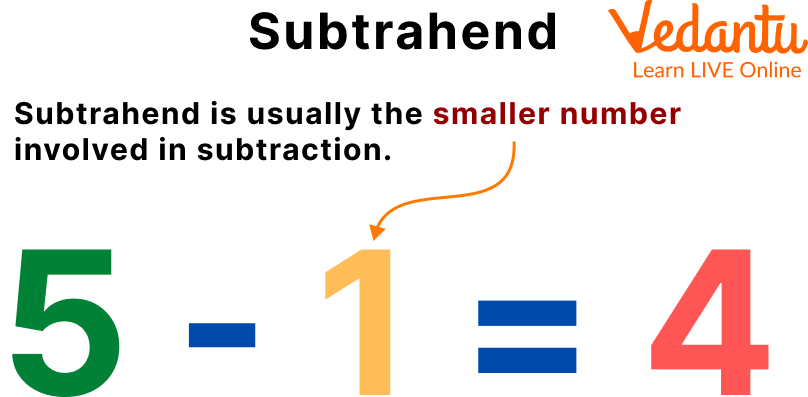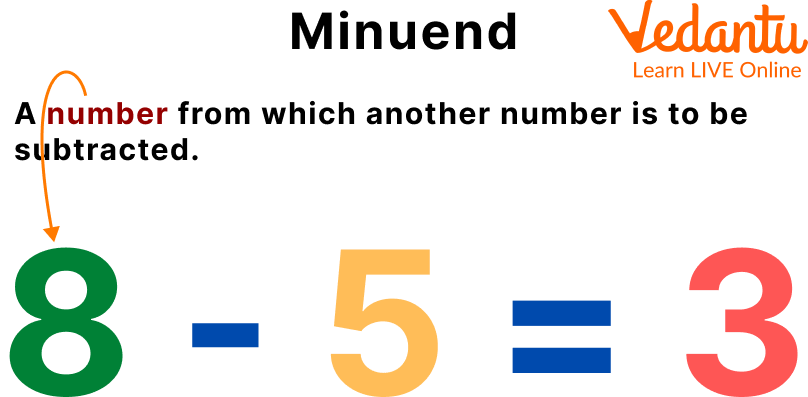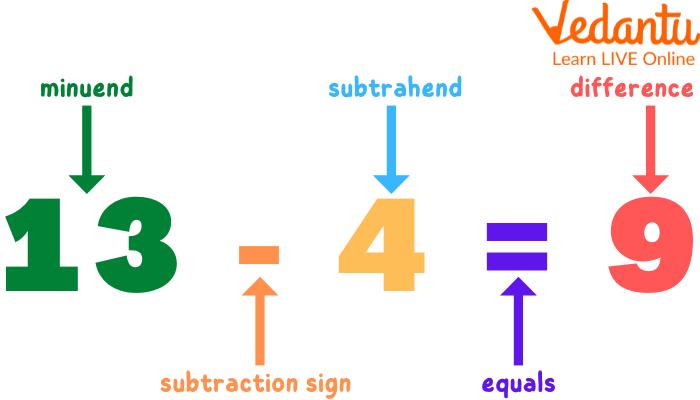Courses
Courses for Kids
Free study material
Free LIVE classes
More

# Subtrahend and Minuend - What They MeanLIVE
Join Vedantu’s FREE Mastercalss

## What is Minuend and Subtrahend?

Subtraction is the process of taking one number away from another. The numbers involved in subtraction have specific, fancy-sounding names to make the whole process much simpler. In this article, we’re learning the basics of these various names and understanding them better to ace subtraction in various forms. We are going to be dealing with minute subtrahend differences. Let’s dive right in.Subtrahend, Minuend, and Difference

## What is Subtrahend?

Before you panic hearing this complex word, you already know these concepts by different names.

Subtrahend is usually the smaller number involved in subtraction. You might know this number as the ‘subtract’ number as you may have referred to it like that in lower classes.

Given below is a Subtrahend example

“Subtract 4 from 20”

4 is the Subtrahend or the ‘subtract’ number (called so as the word “subtract” is present before the number.)

For example, \$3002 - 2000 = 1002\$

Here, 2000 is the smaller number and hence the subtrahend in the given caseSubtrahend

## What is Minuend?

The minuend is usually the bigger number involved in subtraction. You might know this number as the ‘from’ number as you may have referred to it like that in lower classes.

Given below is a minuend example

“Subtract 4 from 20”

20 is the Minuend or the ‘from’ number (called so as the word “from” is present before the number.)

For example, \$3000 - 2000 = 1000\$

Here, 3000 is the bigger number and hence the minuend in the given case.Minuend

## What is the Difference?

The answer of subtraction also has a fancy name, known as the difference.

What you obtain by subtracting the Subtrahend from the Minuend is called the Difference (It is kind of a mouthful but you will get a hang of it)

If you know what a sum is, the difference is pretty equivalent to that. To make it easier to understand, the difference can often be compared to the distance between any two given numbers (minuend and subtrahend).

For example, \$3000 - 2000 = 1000\$

Here, 1000 is the difference between the two numbers and hence the difference in the given case.Difference

## Solved Examples

Q1. Identify the Subtrahend, minuend, and difference in the following problem \$200 - 40 =160\$

Ans:

• 200 is the Minuend as it is the larger number

• 40 is the subtrahend as it is the smaller number

• 160 is found to be the difference

Q2. Write the equation for the following statement and identify the subtrahend, minuend, and difference.

“From 400 subtract 250”

Ans: The above statement can be written as follows: \$400 - 250 = 150\$

• Here, 400 is the minuend

• 250 is the subtrahend

• 150 is found to be the difference

Q3. Identify the Subtrahend, minuend, and difference in the following problem \$500 - 50 = 450\$

Ans:

• 500 is the Minuend as it is the larger number

• 50 is the subtrahend as it is the smaller number

• 450 is found to be the difference

Q4. Write the equation for the following statement and identify the subtrahend, minuend, and difference. “From 750 subtract 100”

Ans: The above statement can be written as follows: \$750 - 100 = 650\$

• Here, 750 is the minuend

• 100 is the subtrahend

• 650 is found to be the difference

Q5. Write the equation for the following statement and identify the subtrahend, minuend, and difference. “From 2000 subtract 500”

Ans: The above statement can be written as follows: \$2000 - 500 = 1500\$

• Here, 2000 is the minuend

• 500 is the subtrahend

• 1500 is found to be the difference

## Practice Problems

Q1. Identify the subtrahend, minuend, and difference from the following, \$300 - 30 = 270\$

Ans: 300-Minuend, 30-Subtrahend, 270-Difference

Q2. Identify the subtrahend, minuend, and difference from the following, \$500 - 80 = 420\$

Ans: 500-Minuend, 80-Subtrahend, 420-Difference

Q3. Arrange the given digits as minuend and subtrahends and give the difference: 4000, 200

Ans: \$4000 - 200 = 3800\$, 3800-difference

Q4. Subtract 500 from 2500 and identify the parts of a subtraction

Ans: 2500-Minuend, 500-Subtrahend. Difference- 2000

Q5. Subtract 200 from 1000 and identify the parts of a subtraction

Ans: 1000-Minuend, 200-Subtrahend. Difference- 800

## Summary

To quickly brush through what we have learned, Minuend is the bigger number of subtraction, also called the ‘from’ number. It usually is the first number in a subtraction problem. Subtrahend is the smaller number of subtraction, also called the ‘subtract’ number. It usually is the second number in a subtraction problem. The difference is the answer to the subtraction problem. It is also referred to as the distance between the given two numbers (minuend and subtrahend).

Last updated date: 01st Oct 2023
Total views: 116.1k
Views today: 4.16k

## FAQs on Subtrahend and Minuend - What They Mean

1. Is Minuend always the bigger number in subtraction?

At beginner levels, minuend is always the bigger number to ensure that the result of the difference obtained is always a positive number. This could also be said like how the subtrahend is always the smaller number. This isn’t always true.

In the case of subtraction of integers where even negative numbers are used, the rule of minuend being the bigger number reverses. As in this case, negative answers (differences with a minus symbol is called negative answer) is usually allowed. So the value of minuend and subtrahend can change too.

2. When is the difference zero in relation to minuend and subtrahend?

When minuend and subtrahend are equal, the difference is zero.

3. Where did the name subtrahend, minuend, and difference originate from?

The story of where words originated from is a pretty interesting one. The word Subtrahend has a Latin origin. Subtrahend originates from ‘Subtrahend’ meaning “to be taken away” or something that is removed. The word Minuend has a Latin origin as well. Originating from the Latin word ‘Minuendus’ meaning diminishing. A minuend is essentially a number whose value is decreasing. The difference originates from the French word meaning Differentia which slowly evolved into the word difference which is often defined as the distance between the 2 numbers.